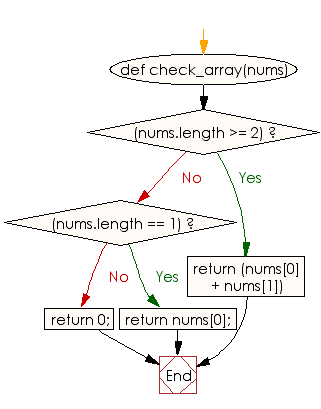﻿ Ruby Array exercises: Create an array of length 2 containing their middle elements from two given arrays of integers of length 3 - w3resource# Ruby Array Exercises: Create an array of length 2 containing their middle elements from two given arrays of integers of length 3

## Ruby Array: Exercise-15 with Solution

Write a Ruby program to create an array of length 2 containing their middle elements from two given arrays of integers of length 3.

Ruby Code:

``````def check_array(nums)
if(nums.length >= 2)
return (nums + nums)
end
if(nums.length == 1)
return nums;
end
return 0;

end
print check_array([1, 2, 5]),"\n"
print check_array([4, 2, 3]),"\n"
print check_array()
``````

Output:

```[2, 5]
[5, 8]
[2, 14]
```

Flowchart:Ruby Code Editor: